# Hierarchical Archimax Copulas

#### 2020-12-11

library(copula) # for hierarchical frailties, generators etc.
library(mev) # for rmev()

### Setup and auxiliary functions

The (frozen) parameters considered are as follows.

## Parameters
d. <- c(2, 3) # copula sector dimensions
d <- sum(d.) # copula dimension
stopifnot(d >= 3) # for 3d plots
n <- 1000 # sample size

We also implement an auxiliary function to generated the hierarchical frailties involved.

##' @title Generated two hierarchical frailties (V0, V01, V02)
##' @param n sample size
##' @param family copula family
##' @param tau Kendall's tau
##' @return 3-column matrix containing the hierarchical frailties
##' @author Marius Hofert and Avinash Prasad
rV012 <- function(n, family, tau)
{
stopifnot(n >= 1, is.character(family), length(tau) == 3, tau > 0)
cop <- getAcop(family) # corresponding AC
th <- iTau(cop, tau = tau) # copula parameters
V0 <- cop@V0(n, theta = th) # sample frailties V_0
V01 <- cop@V01(V0, theta0 = th, theta1 = th) # sample frailties V_01
V02 <- cop@V01(V0, theta0 = th, theta1 = th) # sample frailties V_02
cbind(V0  = V0, V01 = V01, V02 = V02)
}

And a short helper function for plotting (possibly to PDF).

## Plot with possible export to PDF
mypairs <- function(x, pch = ".", file = NULL, width = 6, height = 6, ...)
{
opar <- par(pty = "s")
on.exit(par(opar))
doPDF <- !is.null(file)
if(doPDF) {
pdf(file = file, width = width, height = height)
stopifnot(require(crop))
}
pairs2(x, pch = pch, ...)
if(doPDF) dev.off.crop(file)
}

## 1 ACs vs AXCs vs NACs vs (different) HAXCs

In this example, we compare Archimedean copulas (ACs), Archimax copulas (AXCs), nested Archimedean copulas (NACs) and hierarchical Archimax copulas (HAXCs) with hierarchical frailties only, with hierarchical frailties and hierarchical extreme-value copula (HEVC) but both of the same hierarchical structure, and with hierarchical frailties and HEVC but of different hierarchical structure.

### 1.1 AC (Clayton copula)

To begin with, we sample from a standard Clayton copula.

## Sample frailties (to recycle the frailties, we apply the Marshall--Olkin (MO)
## algorithm manually here)
tau <- 0.4 # Kendall's tau
family <- "Clayton" # frailty family
cop <- getAcop(family) # corresponding AC
th <- iTau(cop, tau = tau) # copula parameter
set.seed(271) # for reproducibility
V <- cop@V0(n, theta = th) # sample frailty
E <- matrix(rexp(n * d), ncol = d) # sample Exp(1)
U.AC <- cop@psi(E/V, theta = th) # MO

## Plot
mypairs(U.AC)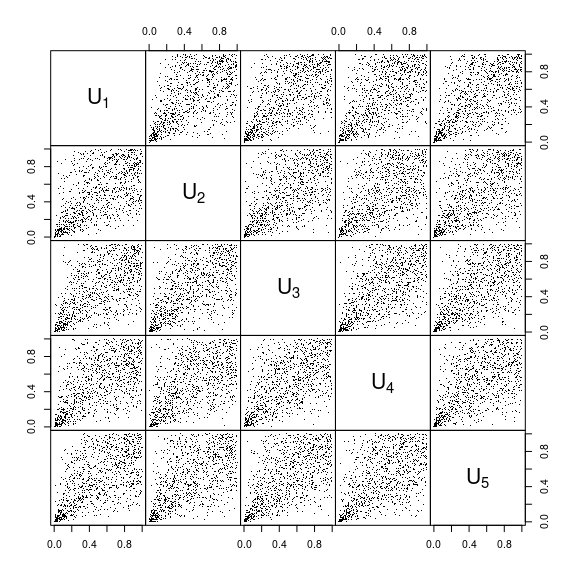### 1.2 AXC (Clayton frailties and Gumbel EVC)

Now we sample from an Archimax copula with gamma frailties (as underlying the Clayton family; we recycle the frailties from 1.1) and Gumbel EVC (parameters chosen such that Kendall’s tau equals 0.5).

## Generate Gumbel EVC sample with Exp(1) margins
tau.EVC <- 0.5 # Kendall's tau
family.EVC <- "Gumbel" # EVC family
th.EVC <- iTau(getAcop(family.EVC), tau = tau.EVC) # EVC parameter
cop.EVC <- onacopulaL(family.EVC, list(th.EVC, 1:d)) # EVC
set.seed(271) # for reproducibility
U.EVC <- rCopula(n, copula = cop.EVC) # sample EVC
E.EVC <- -log(U.EVC) # map the EVC to Exp(1) margins

## Combine with the (same) Clayton frailties (as before)
U.AXC <- cop@psi(E.EVC/V, theta = th)

## Plot
mypairs(U.AXC)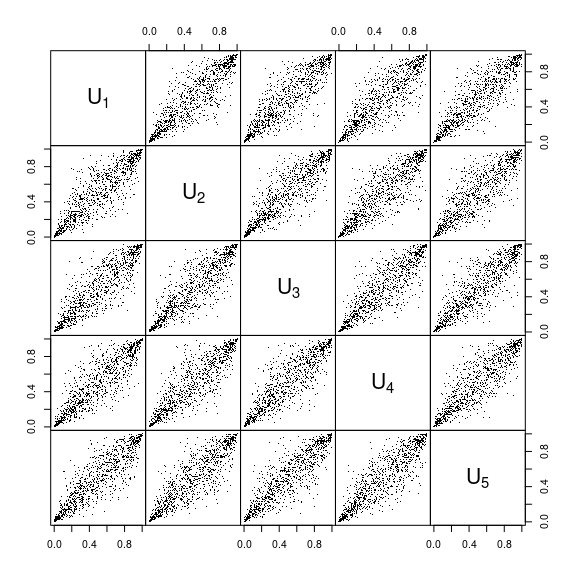### 1.3 NAC (nested Clayton)

Sampling from a NAC based on Clayton’s family with parameters such that Kendall’s tau equals 0.2 between the two sectors, 0.4 within the first sector and 0.6 within the second sector, can be done as follows.

## Generate hierarchical frailties
tau.N <- c(0.2, 0.4, 0.6) # Kendall's taus
family.N <- "Clayton" # frailty family
cop.N <- getAcop(family.N) # corresponding AC
th.N <- iTau(cop.N, tau = tau.N) # copula parameters
set.seed(271) # for reproducibility
V.N <- rV012(n, family = family.N, tau = tau.N) # generate corresponding frailties
V0  <- V.N[,"V0"]
V01 <- V.N[,"V01"]
V02 <- V.N[,"V02"]

## Combine with independent Exp(1)
U.NAC <- cbind(cop.N@psi(E[,1:d.]/V01,     theta = th.N),
cop.N@psi(E[,(d.+1):d]/V02, theta = th.N))

## Plot
mypairs(U.NAC)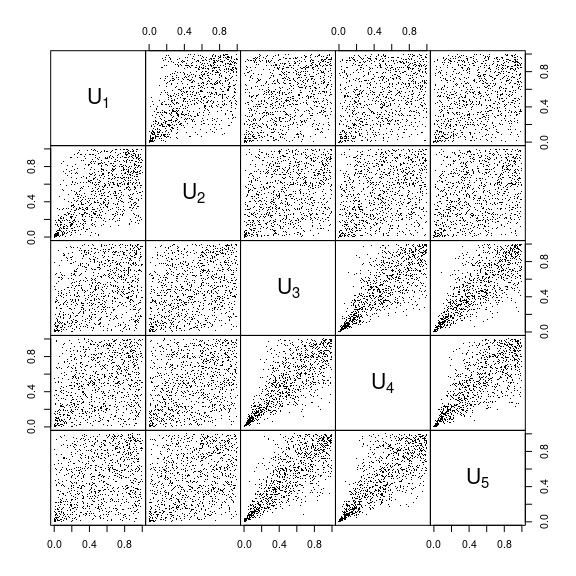### 1.4 HAXC (hierarchical Clayton frailties and Gumbel EVC)

Sampling from a NAXC based on hierarchical Clayton frailties (recycled from 1.3) and Gumbel EVC (realizations recycled from 1.2). Note that hierarchies only take place at the levels of the frailties in this case.

## Generate samples
U.HAXC <- cbind(cop.N@psi(E.EVC[,1:d.]/V01,     theta = th.N),
cop.N@psi(E.EVC[,(d.+1):d]/V02, theta = th.N))

## Plot
mypairs(U.HAXC)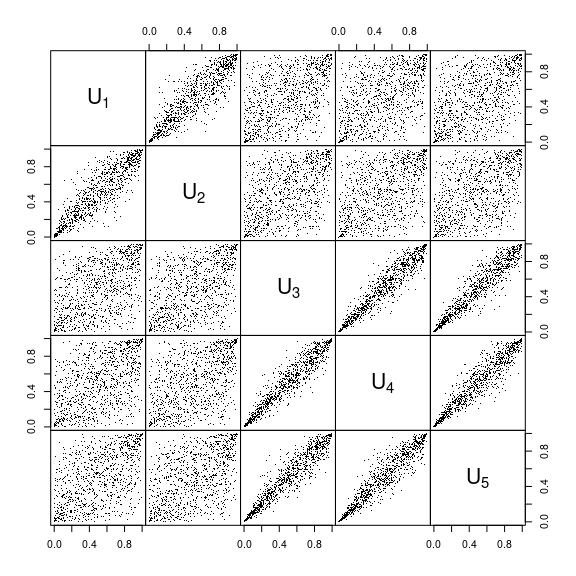### 1.5 HAXC (hierarchical Clayton frailties and hierarchical Gumbel EVC, same hierarchical structure)

After this warm-up, we can consider sampling from a HAXC based on hierarchical Clayton frailties (recycled from 1.3) and a hierarchical Gumbel EVC (with sector sizes 2 and 3 and parameters such that Kendall’s tau equals 0.2 between the two sectors, 0.5 within the first sector and 0.7 within the second sector). Note that there are two types of hierarchies involved, at the level of the (hierarchical) frailties and at the level of the (hierarchical) EVC. Furthermore, their hierarchical structure is the same.

## Sampling from a hierarchical Gumbel copula
tau.HEVC <- c(0.2, 0.5, 0.7) # Kendall's taus
family.HEVC <- "Gumbel" # EVC family
cop.HEVC <- getAcop(family.HEVC) # corresponding base EVC
th.HEVC <- iTau(cop.HEVC, tau = tau.HEVC) # copula parameters
cop.HEVC <- onacopulaL(family.HEVC, list(th.HEVC, NULL,
list(list(th.HEVC, 1:d.),
list(th.HEVC, (d.+1):d)))) # hierarchical EVC
set.seed(271) # for reproducibility
U.HEVC <- rCopula(n, copula = cop.HEVC) # sample the HEVC
E.HEVC <- -log(U.HEVC) # map the HEVC samples to Exp(1) margins

## Combine the hierarchical Gumbel EVC with Exp(1) margins with the hierarchical frailties
U.HAXC.HEVC.same <- cbind(cop.N@psi(E.HEVC[,1:d.]/V01,     theta = th.N),
cop.N@psi(E.HEVC[,(d.+1):d]/V02, theta = th.N))

## Plot
mypairs(U.HAXC.HEVC.same)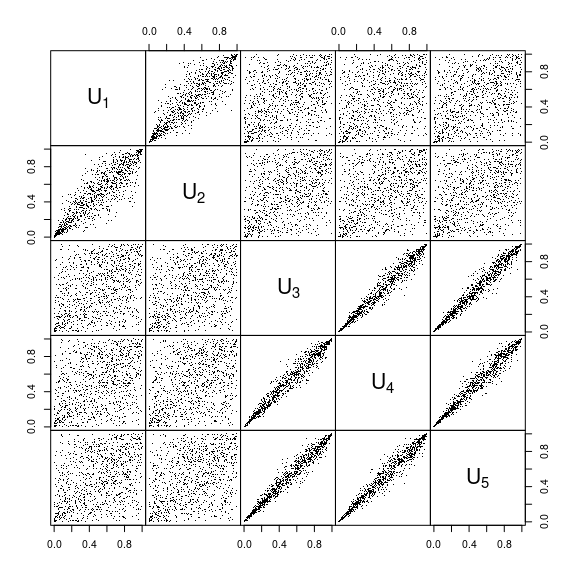### 1.6 HAXC (hierarchical Clayton frailties and hierarchical Gumbel EVC, different hierarchical structure)

Sampling from a HAXC (recycling realizations from 1.5) for which the hierarchical structures of the hierarchical frailties (sector sizes 3 and 2, respectively) and of the hierarchical Gumbel EVC (sector sizes 2 and 3, respectively) differ.

## Combine the hierarchical Gumbel EVC with Exp(1) margins with the hierarchical frailties
## in an 'asymmetric' way (hierarchical structures of the HEVC and the hierarchical frailties differ)
d.. <- rev(d.) # 3, 2
U.HAXC.HEVC.dffr <- cbind(cop.N@psi(E.HEVC[,1:d..]/V01,     theta = th.N), # first three components get V01
cop.N@psi(E.HEVC[,(d..+1):d]/V02, theta = th.N)) # last two components get V02

## Plot
mypairs(U.HAXC.HEVC.dffr)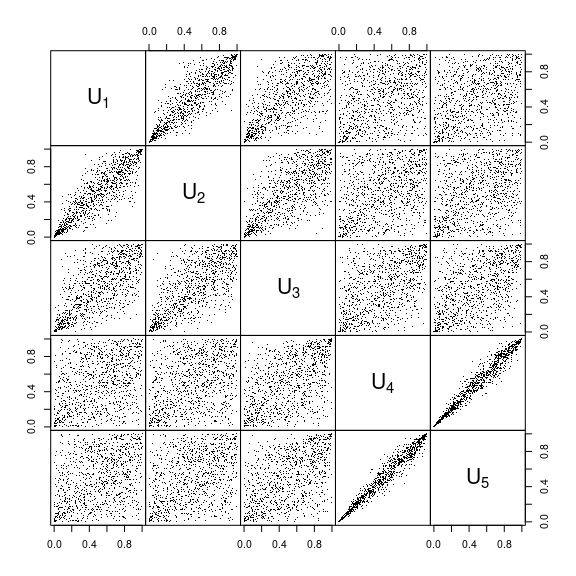## 2 EVCs vs HEVCs vs (different) HAXCs

Again in terms of an example, we now compare extreme-value copulas (EVCs), hierarchical extreme-value copulas (HEVCs) and hierarchical Archimax copulas (HAXCs) with single frailty and HEVC, with hierarchical frailties and EVC, with hierarchical frailties and HEVC but both of the same hierarchical structure, and with hierarchical frailties and HEVC but of different hierarchical structure.

### 2.1 EVC

To begin with, we draw $$n$$ samples from an extremal t copula with $$\nu=3.5$$ degrees of freedom and off-diagonal entry of the correlation matrix parameter $$P$$ to be 0.7.

## Correlation matrix (parameter matrix of the extremal t)
P <- matrix(0.7, ncol = d, nrow = d)
diag(P) <- 1

## Extremal t copula (EVC)
nu <- 3.5
set.seed(271)
U.EVC <- exp(-1/rmev(n, d = d, param = nu, sigma = P, model = "xstud")) # apply unit Frechet margins to get U

## Plot
mypairs(U.EVC)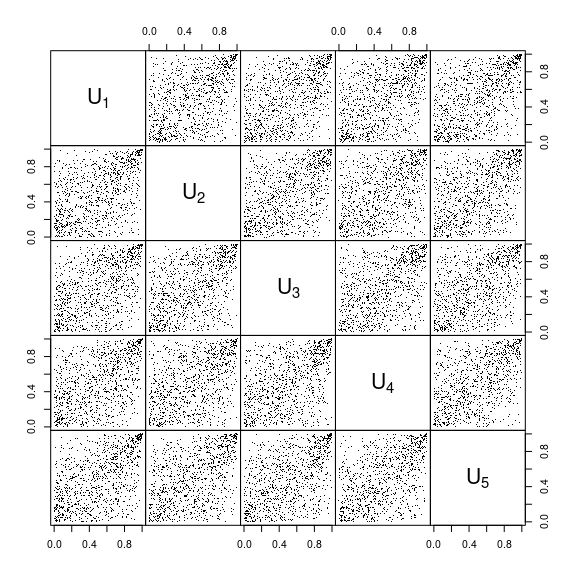### 2.2 HEVC

Now, we sample from an hierarchical extremal t copula with two sectors of sizes 2 and 3 such that the correlation matrix $$P$$ has entries 0.2 for pairs belonging to different sectors, 0.5 for pairs belonging to the first sector and 0.7 for pairs belonging to the second sector.

## Construct a hierarchical correlation matrix for the extremal t
P.h <- matrix(0.2, ncol = d, nrow = d)
P.h[1:d., 1:d.] <- 0.5
P.h[(d-d.+1):d, (d-d.+1):d] <- 0.7
diag(P.h) <- 1

## Hierarchical extremal t copula (HEVC)
X.et <- rmev(n, d = d, param = nu, sigma = P.h, model = "xstud")
U.HEVC <- exp(-1/X.et) # apply unit Frechet margins to get U

## Plot
mypairs(U.HEVC)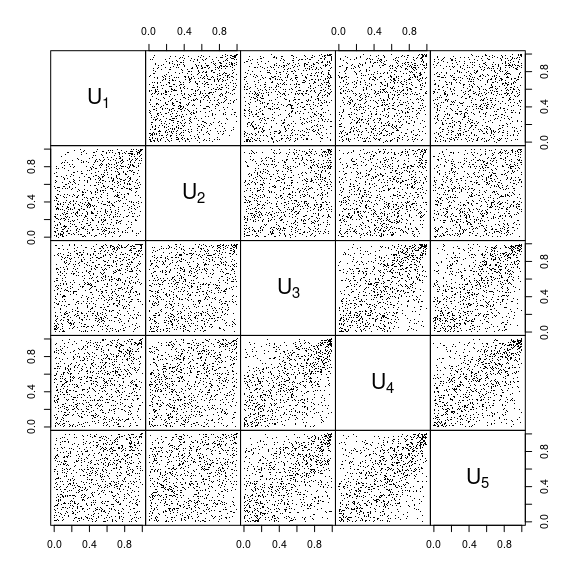### 2.3 HAXC (Clayton frailty and HEVC)

Sampling from a HAXC with Clayton frailty (recycled from 1.1) and hierarchical extremal t copula (on top of the unit exponentials; recycled from 2.2) can be done as follows.

## Construct samples
E.HEVC <- -log(U.HEVC) # map to Exp(1) margins
U.AXC.HEVC <- cop@psi(E.HEVC/V, theta = th)

## Plot
mypairs(U.AXC.HEVC)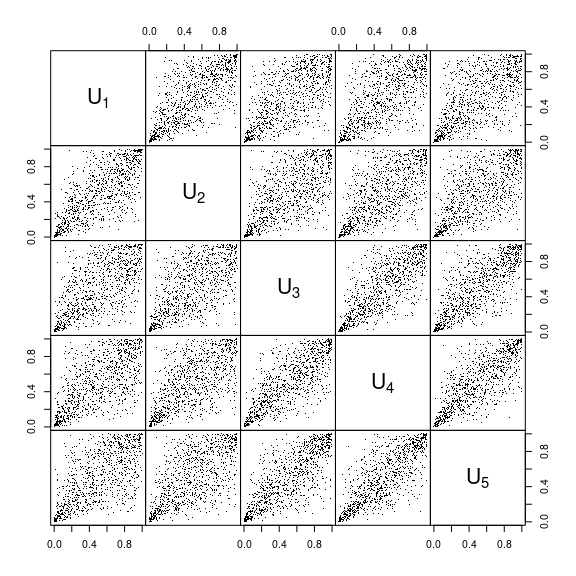### 2.4 HAXC (hierarchical Clayton frailties and EVC)

Consider a HAXC with hierarchical Clayton frailties (recycled from 1.3) and extremal t EVC (on top of the unit exponentials; recycled from 2.1).

## Construct samples
E.EVC <- -log(U.EVC)
U.HAXC.EVC <- cbind(cop.N@psi(E.EVC[,1:d.]/V01,     theta = th.N),
cop.N@psi(E.EVC[,(d.+1):d]/V02, theta = th.N))

## Plot
mypairs(U.HAXC.EVC)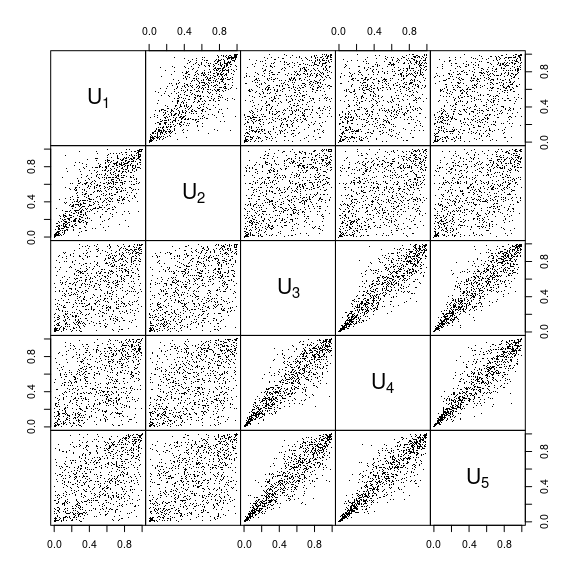### 2.5 HAXC (hierarchical Clayton frailties and HEVC, same hierarchical structure)

Now consider a HAXC with hierarchical Clayton frailties (recycled from 1.3) and hierarchical extremal t EVC (on top of the unit exponentials; recycled from 2.2 and 2.3). Note that there are two types of hierarchies involved, at the level of the (hierarchical) frailties and at the level of the (hierarchical) EVC.

## Construct samples
U.NAXC.HEVC.same <- cbind(cop.N@psi(E.HEVC[,1:d.]/V01,     theta = th.N),
cop.N@psi(E.HEVC[,(d.+1):d]/V02, theta = th.N))

## Plot
mypairs(U.HAXC.HEVC.same)### 2.6 HAXC (hierarchical Clayton frailties and HEVC, different hierarchical structure)

Sampling from a HAXC (recycling realizations from 2.5) for which the hierarchical structures of the frailties (sector sizes 3 and 2, respectively) and of the HEVC (sector sizes 2 and 3, respectively) differ in this case.

## Construct samples
U.NAXC.HEVC.dffr <- cbind(cop.N@psi(E.HEVC[,1:d..]/V01,     theta = th.N), # first three components get V01
cop.N@psi(E.HEVC[,(d..+1):d]/V02, theta = th.N)) # last two components get V02

## Plot
mypairs(U.HAXC.HEVC.dffr)